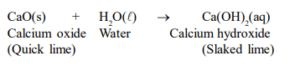# A solution of a substance ' $X$ ' is used for white washing.Question.

A solution of a substance ' $X$ ' is used for white washing.

(i) Name the substance ' $X$ ' and write its formula.

(ii) Write the reaction of the substance 'X' with water.

solution:

(i) The substance ' $\mathrm{X}$ ' is calcium oxide. Its chemical formula is $\mathrm{CaO}$.

(ii) Calcium oxide reacts vigorously with water to form calcium hydroxide (slaked lime).